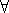## CGAL::Random_points_in_square_2<Point_2, Creator>

### Definition

The class Random_points_in_square_2<Point_2, Creator> is an input iterator creating points uniformly distributed in a half-open square. The default Creator is Creator_uniform_2<Kernel_traits<P>::Kernel::RT,P>.

### Is Model for the Concept

InputIterator
PointGenerator

#include <CGAL/point_generators_2.h>

### Types

 typedef std::input_iterator_tag iterator_category; typedef Point_2 value_type; typedef std::ptrdiff_t difference_type; typedef const Point_2* pointer; typedef Point_2 reference;

### Operations

 Random_points_in_square_2 g ( double a, Random& rnd = default_random);
g is an input iterator creating points of type Point_2 uniformly distributed in the half-open square with side length 2 a, centered at the origin, i.e.p = *g: -ap.x() < a and -ap.y() < a . Two random numbers are needed from rnd for each point.